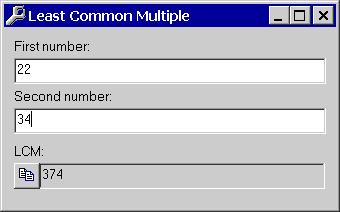LCM and GCF Calculator

The image shown is the LCM (Least Common Multiple) calculator, which allows the user to calculate the LCM of any two numbers. There is also a GCF (Greatest Common Factor) calculator which works in the same fashion.Math tools included in the Mega Toolbox are:
• Least Common Multiple and Greatest Common Factor calculator
• Base converter
• Happy Number Calculator
• Polar and Rectangular Calculator
• Prime Factorization Calculator